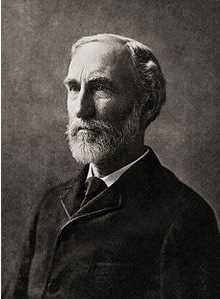Search:

# Gibbs free energy (free enthalpy)Josiah Willard Gibbs (1839-1903)

## Définition

In order to bring together the three criteria od spontaneity (decrease of energy, high temperature for endothermic reactions, low temperature for exothermic reactions, trend to disorder) , Willard Gibbs introduced a new state function called free energy or free enthalpy (symbol $G$, unit Joule $J$) :

Definition of free energy of a system: $G$ $=$ $H$ $-$ $T\cdot S$ $H$: enthalpy $S$: entropy $T$: absolute temperature

A system passing at temperature $T$ from an initial state to a final state undergoes a variation of free energy given by: $\Delta G$ $=G_f$ $-$ $G_i$ $=H_f$ $-$ $T\cdot S_f$ $-$ $(H_i$ $-$ $T\cdot S_i)$ $=H_f$ $-$ $H_i$ $-$ $T(S_f$ $-$ $S_i)$

Transformation at constant temperature $T$: $\Delta G$ $=$ $\Delta H$ $-$ $T\cdot \Delta S$

## The spontaneity of transformations

Gibbs established the following rule:

A transformation is spontaneous if $\Delta G\lt 0$ or otherwise: $\Delta H$ $-$ $T\cdot \Delta S$ $<$ $0$

In an isolated system ($\Delta H$ $=$ $0$), this rule can be simplified: $-T\cdot \Delta S$ $\lt 0$ $T\cdot \Delta S$ $\gt 0$ $\Delta S \gt 0$, because $T \gt 0$ and so we obtain the second principle of thermodynamics

## Examples

### Example 1

$N_2O_4(g)$ $\longrightarrow$ $2NO_2(g)$ $\Delta S=175.8\frac{J}{K\cdot mol}$ $\Delta H=57.2kJ$ $T=298.15K$ $\Delta G$ $=$ $\Delta H$ $-$ $T\cdot \Delta S$ $=$ $4.8 kJ$ $\Delta G \gt 0$ At $25^oC$, this reaction is not spontaneous, but a rise in temperature $T$ promotes the term $-T\cdot \Delta S$ and $\Delta G$ can be rendered negative, and so the reaction will be spontaneous.

### Example 2

Formation of iron(III) oxide from metallic iron has a (negative !) variation of entropy equal to $-272\frac{J}{K\cdot mol}$. $2Fe(s)$ $+$ $\frac{3}{2}O_2$ $\longrightarrow$ $Fe_2O_3(s)$ $\Delta H$ $=$ $\Delta H_f(Fe_2O_3(s))$ $=$ $-824.2kJ$ $T$ $=$ $298.15K$ $\Delta G$ $=$ $\Delta H$ $-$ $T\cdot \Delta S$ $=$ $-743 kJ$ $\Delta G\lt 0$ At $25^oC$, this reaction is spontaneous.

### Example 3

$C_5H_{12}(l)$ $+$ $11O_2(g)$ $\rightarrow$ $5CO_2(g)$ $+$ $6H_2O(g)$ This reaction is a combustion, so it liberates heat at constant pressure: $\Delta H\lt 0$. The reaction produces a disordered mixture of small molecules, so $\Delta S \gt 0$. Therefore: $T\Delta S \gt 0$ , because $T \gt 0$ $-T\Delta S \lt 0$ $\Delta H$ $-$ $T\Delta S \lt 0$ $\Delta G \lt 0$ and the reaction will be spontaneous.

### Example 3: Photosynthesis

$6CO_2(g)$ $+$ $6H_2O(g)$ $\longrightarrow$ $C_6H_{12}O_{6}(l)$ $+$ $6O_2(g)$ Let's see if this reaction is possible if the reagents are isolated from the surroundings ! The disorder decreases from the left to the right side because 6 molecules of liquid water and 6 gaseous molecules of $CO_2$ are more disordered than one molecule of glucose $C_6H_{12}O_6(aq)$ and 6 gaseous molecules of $O_2$, therefore $\Delta S\lt 0$. For an isolated system ( $\Delta H$ $=$ $0$ ) it follows $\Delta G$ $=$ $\Delta H$ $-$ $T\Delta S \gt 0$ Photosynthesis would not be spontaneous if energy was not coming from the surroundings (sun)!